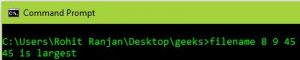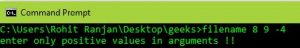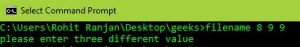# Command line arguments example in C

Prerequisite: Command_line_argument. The problem is to find the largest integer among the three using command line arguments. Notes:

• Command-line arguments are given after the name of the program in the command-line shell of Operating Systems. To pass command line arguments, we typically define main() with two arguments: the first argument is the number of command-line arguments and the second is a list of command-line arguments.
`int main(int argc, char *argv[]) { /* ... */ }`
• atoi – Used to convert string numbers to integers

Examples:

```Input  : filename 8 9 45
Output : 45 is largest

Input  : filename 8 9 9
Output : Two equal number entered

Input  : filename 8 -9 9
Output : negative number entered```

When calling the program, we pass three integers along with its filename, and then the program prints out the largest of the three numbers.

Approach:

1. The program “return 1” if one of the two following conditions is satisfied:
• If any two numbers are the same, print the statement “two equal numbers entered”.
• If any of the numbers is negative, print “negative number entered”.
2. Else “return 0” if three different integers are entered.

For better understanding, run this code for yourself.

Algorithm for this program:

1. Check whether there are 4 arguments or not.
2. Use the ‘atoi’ function to convert argument of the string type to integer types.
3. Check if each number is positive or not and whether there is a difference between them.
4. Use conditional statement to find out the largest number among the all three numbers.

Below is the implemetation of the above algorithm

## C

 `// C program for finding the largest integer` `// among three numbers using command line arguments` `#include ` `#include `   `// Taking argument as command line` `int` `main(``int` `argc, ``char` `*argv[]) ` `{` `    ``int` `a, b, c;`   `    ``// Checking if number of argument is` `    ``// equal to 4 or not.` `    ``if` `(argc < 4 || argc > 5) ` `    ``{` `        ``printf``("enter 4 arguments only eg.\"filename arg1 arg2 arg3!!\"");` `        ``return` `0;` `    ``}`   `    ``// Converting string type to integer type` `    ``// using function "atoi( argument)" ` `    ``a = ``atoi``(argv); ` `    ``b = ``atoi``(argv);` `    ``c = ``atoi``(argv);`   `    ``// Checking if all the numbers are positive or not` `    ``if` `(a < 0 || b < 0 || c < 0) ` `    ``{` `        ``printf``("enter only positive values in arguments !!");` `        ``return` `1;` `    ``}`   `    ``// Checking if all the numbers are different or not` `    ``if` `(!(a != b && b != c && a != c)) ` `    ``{` `        ``printf``("please enter three different value ");` `        ``return` `1;` `    ``}` `    ``else` `    ``{` `        ``// Checking condition for "a" to be largest` `        ``if` `(a > b && a > c)             ` `            ``printf``("%d is largest", a);`   `        ``// Checking condition for "b" to be largest    ` `        ``else` `if` `(b > c && b > a) ` `            ``printf` `("%d is largest", b);`   `        ``// Checking condition for "c" to be largest..` `        ``else` `if` `(c > a && c > b) ` `            ``printf``("%d is largest ",c);` `    ``}` `    ``return` `0;` `}`

Output :### Complexity Analysis :

• Space Complexity is constant as it dos not depend on the size of the input.
• Time Complexity – 0(1) constant as well.

This program is efficient for finding the largest integer among three numbers using command line arguments since it has a very low time and space complexity.

Whether you're preparing for your first job interview or aiming to upskill in this ever-evolving tech landscape, GeeksforGeeks Courses are your key to success. We provide top-quality content at affordable prices, all geared towards accelerating your growth in a time-bound manner. Join the millions we've already empowered, and we're here to do the same for you. Don't miss out - check it out now!